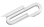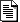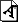Spring 2020

# Tuesday April 7

Schedule ... no Jim on Thursday. Alternatives?

## energy - finish up

Questions about homework or anything else?

## momentum

Sometimes called "linear momentum" (to distinguish it from "angular momentum".)

I would expect the text to cover this here but apparently they wait until the rotations chapter :center of mass (i.e. average over masses position).

related topics :

• collisions (elastic, inelastic)
• rockets and "rocket equation"
• impulse

## the assignment for next Tuesday

... has been posted.

## aside

In quantum physics, the momentum of a particle is related to its wavelength when that particle acts like a wave. This leads to the notion that there is a fundamental uncertainty between position and momentum - that the two concepts cannot be defined exactly for the same particle at once.

In the fundamental math of physics, the symmetry of the equations of motion under spatial translation is what leads to the conservation of momentum.

In a (very) similar way, the energy of a quantum particle is related to its frequency (vibrations per second) when it acts like a wave. That leads to the notion that there is a fundamental uncertainty between energy and time - that the two concepts cannot be defined exactly for the same particle at once.

See for example wikipedia: uncertainty principle

• $$\Delta x \Delta p \ge \hbar /2$$
• $$\Delta t \Delta E \ge \hbar /2$$

where $$\hbar$$ is a constant - Plank's constant over two pi, and the $$\Delta \text{foo}$$ is the uncertainty inherit in trying to measure any quantity foo.

It's interesting that in our starting definitions, space and time show up connected to the other concepts, not to the "complentary" ones in quantum physics.

• dP = F * dt (connecting momentum to force and time)
• dE = F * dx (connecting energy to force and distance)

## in class

I've attached the .txt file and .pdf notepad from my blackboard scrawling.

And I've uploaded a recording of today's meeting; check out the link on the left.

https://cs.marlboro.college /cours /spring2020 /mechanics /notes /apr7
## attachmentslast modified sizecenter_of_mass.txt Sun Oct 17 2021 01:36 pm 960Bcenter_of_mass_notepad.pdf Sun Oct 17 2021 01:36 pm 341K